## How to Calculate and Solve for Dependent Events | Probability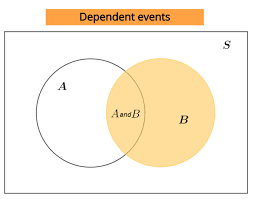The image above represents dependent events.

To compute for dependent events, four essential parameters are needed and these parameters are Number of Times Event A can occur (xA), Number of Times Event B can occur (xB) and Total Number of All Possible Outcomes (N).

The formula for calculating independent events:

P(A and B) = P(A) x P(B|A)

Where;

P(A and B) = Dependent events
xA = Number of Times Event A can occur
xB = Number of Times Event B can occur
N = Total Number of All Possible Outcomes
P(A) = xAN
P(B|A) = xB(N – 1)

Let’s solve an example;
Find the dependent events when the number of times event A can occur is 8, number of times event B can occur is 11 and the total number of all possible outcomes is 18.

This implies that;

xA = Number of Times Event A can occur = 8
xB = Number of Times Event B can occur = 11
N = Total Number of All Possible Outcomes = 18

P(A and B) = P(A) x P(B|A)
P(A and B) = xAN x xB(N – 1)
P(A and B) = 818 x 1117
P(A and B) = (8)(11)(18)(17)
P(A and B) = 88306
Dividing the numerator and denominator by 2
P(A and B) = 44153
P(A and B) = 0.287

Therefore, the dependent events is 0.287.

## How to Calculate and Solve for Independent Events | Probability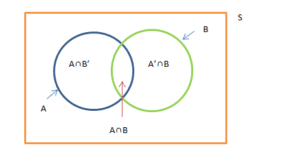The image above represents independent events.

To compute for independent events, four essential parameters are needed and these parameters are Number of Times Event A can occur (xA), Number of Times Event B can occur (xB) and Total Number of All Possible Outcomes (N).

The formula for calculating independent events:

P(A and B) = P(A) x P(B)

Where;

P(A and B) = Independent events
xA = Number of Times Event A can occur
xB = Number of Times Event B can occur
N = Total Number of All Possible Outcomes
P(A) = xAN
P(B) = xBN

Let’s solve an example;
Find the independent events when the number of times event A can occur is 11, number of times event B can occur is 15 and the total number of all possible outcomes is 22.

This implies that;

xA = Number of Times Event A can occur = 11
xB = Number of Times Event B can occur = 15
N = Total Number of All Possible Outcomes = 22

P(A and B) = P(A) x P(B)
P(A and B) = xAN x xBN
P(A and B) = 1122 x 1522
P(A and B) = (11)(15)(22)(22)
P(A and B) = 165484
Dividing the numerator and denominator by 11
P(A and B) = 1544
P(A and B) = 0.3409

Therefore, the independent events is 0.3409.

## How to Calculate and Solve for Impossibility | Probability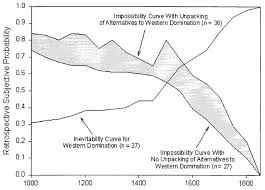The image above represents impossibility.

To compute for impossibility, one essential parameter is needed and this parameter is Total Number of All Possible Outcomes (N).

The formula for calculating impossibility:

P(A) = x N

Where;

P(A) = Imposssibility
N = Total Number of All Possible Outcomes

Let’s solve an example;
Find the impossibility when the total number of all possible outcomes is 12.

This implies that;

N = Total Number of All Possible Outcomes = 12

P(A) = x N
P(A) = 0 12
P(A) = 0

Therefore, P(impossibility) is 0.

## How to Calculate and Solve for P(not A) | Probability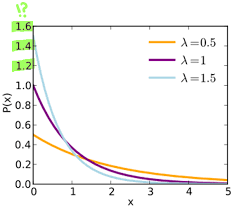The image above represents P(not A).

To compute for P(not A), two essential parameters are needed and these parameters are Number of Times in Which Event A Can Occur (x) and Total Number of All Possible Outcomes (N).

The formula for calculating P(not A):

P(not A) = (N – x)N

Where;

x = Number of Times in Which Event A can Occur
N = Total Number of All Possible Outcomes

Let’s solve an example;
Find the P(not A) when the number of times in which event A can occur is 7 and the total number of all possible outcomes is 11.

This implies that;

x = Number of Times in Which Event A can Occur = 7
N = Total Number of All Possible Outcomes = 11

P(not A) = (N – x) N
P(not A) = (11 – 7) 11
P(not A) = 4 11
P(not A) = 0.36

Therefore, the P(not A) is 0.36.

## How to Calculate and Solve for P(A) | Probability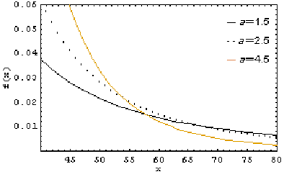The image above represents P(A).

To compute for P(A), two essential parameters are needed and these parameters are Number of Times in Which Event A Can Occur (x) and Total Number of All Possible Outcomes (N).

The formula for calculating P(A):

P(A) = x N

Where;

x = Number of Times in Which Event A can Occur
N = Total Number of All Possible Outcomes

Let’s solve an example;
Find the P(A) when the number of times in which event A can occur is 10 and the total number of all possible outcomes is 22.

This implies that;

x = Number of Times in Which Event A can Occur = 10
N = Total Number of All Possible Outcomes = 22

P(A) = x N
P(A) = 10 22
Dividing the numerator and denominator by 2
P(A) = 5 11
P(A) = 0.45

Therefore, the P(A) is 0.45.

## How to Calculate and Solve for Certainty | Probability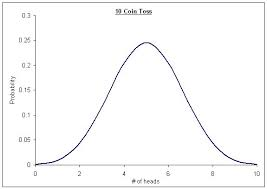The image above represents Certainty.

To compute for certainty, one essential parameter is needed and this parameter is Total Number of All Possible Outcomes (N).

The formula for calculating Certainty:

P(A) = x N = N N

Where;

P(A) = Certainty
N = Total Number of All Possible Outcomes = 2

Let’s solve an example;
Given that the total number of all possible outcomes is 2. Find the Certainty?

This implies that;

N = Total Number of All Possible Outcomes = 2

P(A) = x N = N N
P(A) = 2 2
P(A) = 1

Therefore, P(certainty) is 1.